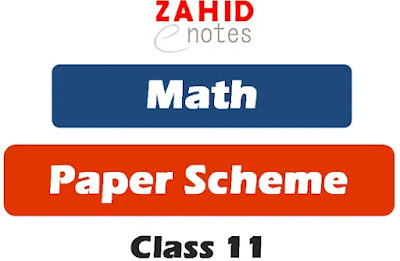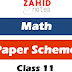IWe have updated 1st year pairing scheme math 2022 at Zahid Notes. Well, this is not the time to write details of everything. As you will skip all these texts and go to pairing scheme instead. So, these are the texts to fill the post and to have something written along the paper scheme of 1st year maths 2022.

# 1st year pairing scheme Math 2022 Punjab Board

You should see:

3. 1st year paper patterns

Zahid Notes is an educational website leading the niche. We provide every help to the students and the teacher across Pakistan and in the world.

As there are others who publish good online content, I appreciate the efforts of those people. But there are website that are scams and have not content but they claim that they have content.

Avoid those websites. The 1st year scheme of maths is not given below and download it in pdf if you want.

## 1st year class 11 Math paper scheme 2022 downloadQuestion No. 1 MCQs = 20 (1 from chapter 1, 2, 5, 9, 10, 11, 13, 14 each and 2 mcqs from chapter 3, 4, 6, 7, 8, 12 each)

#### Short questions

Question No. 2 (8/12)
Chapter 1 = 3
Chapter 2 = 3
Chapter 3 = 3
Chapter 4 = 3

Question No. 3 (8/12)
Chapter 5 = 2
Chapter 6 = 5
Chapter 7 = 2
Chapter 8 = 3

Question No. 4 (9/13)
Chapter 9 = 3
Chapter 10 = 3
Chapter 11 = 1
Chapter 12 = 3
Chapter 13 = 1
Chapter 14 = 2

#### Long questions (Attempt ANY THREE questions from FIVE Questions)

Questions No. 5
Chapter 3 = part A
Chapter 4 = Part B

Questions No. 6
Chapter 5 = part A
Chapter 6 = Part B

Questions No. 7
Chapter 67 = part A
Chapter 8 = Part B

Questions No. 8
Chapter 9 = part A
Chapter 10 = Part B

Questions No. 9
Chapter 12 = part A
Chapter 13 = Part B

To download pdf file of all subjects paper scheme of class 11 22, click the download button to download the pdf.

Now you can see I have given you new scheme of 1st year math. Some changes have been made in the new scheme for 2022 exams in all Punjab boards.

1st year pairing scheme Math 2022Reviewed by Saif Ullah ZahidonMay 14, 2022Rating: 5
1.